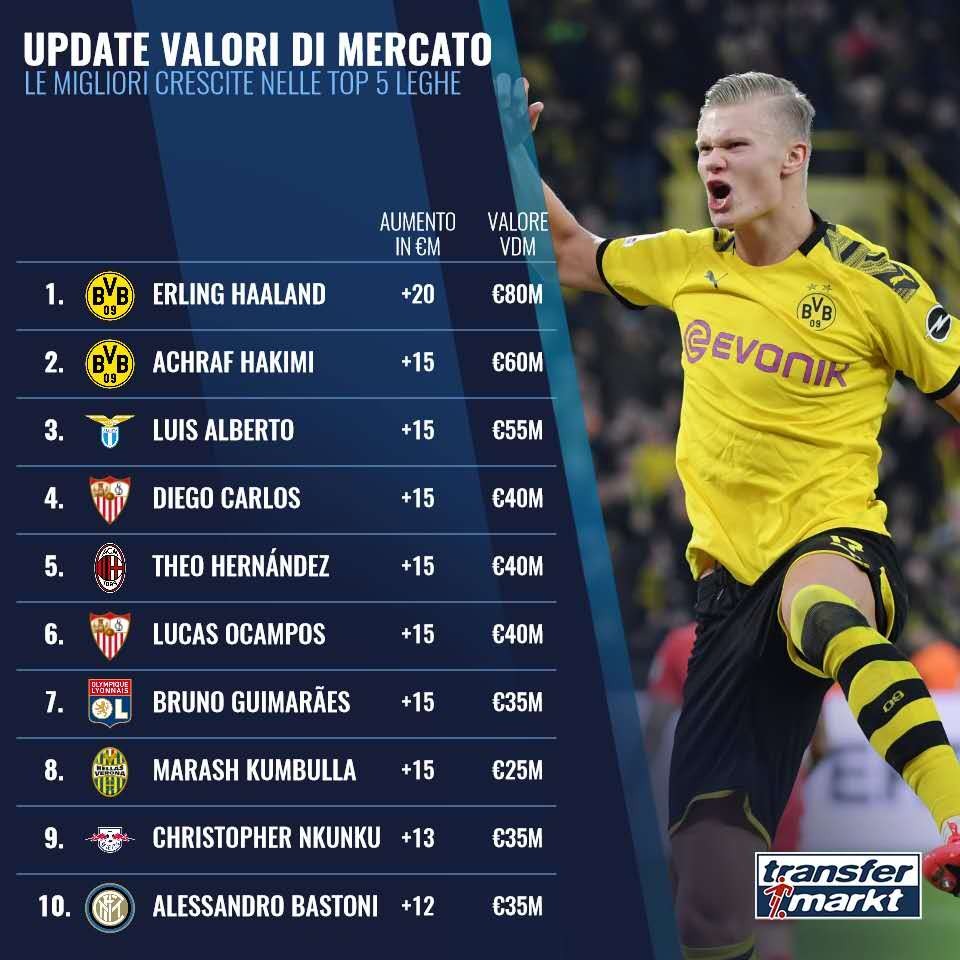1. 哈兰德（多特蒙德）：

6000+2000=8000

2. 哈基米（多特蒙德）：

4500+1500=6000

2. 阿尔贝托（拉齐奥）：

4000+1500=5500

2. 迭戈-卡洛斯（塞维利亚）：

2500+1500=4000

2. 特奥-埃尔南德斯（AC米兰）：

2500+1500=4000

2. 吉马雷斯（里昂）：

2000+1500=3500

2. 库姆布拉（维罗纳）：

1000+1500=2500

9. 恩昆库（莱比锡）：

2200+1300=3500

10. 巴斯托尼（国际米兰）：

2300+1200=3500

(编辑：姚凡)